Ex 10.3

Chapter 10 Class 12 Vector Algebra
Serial order wise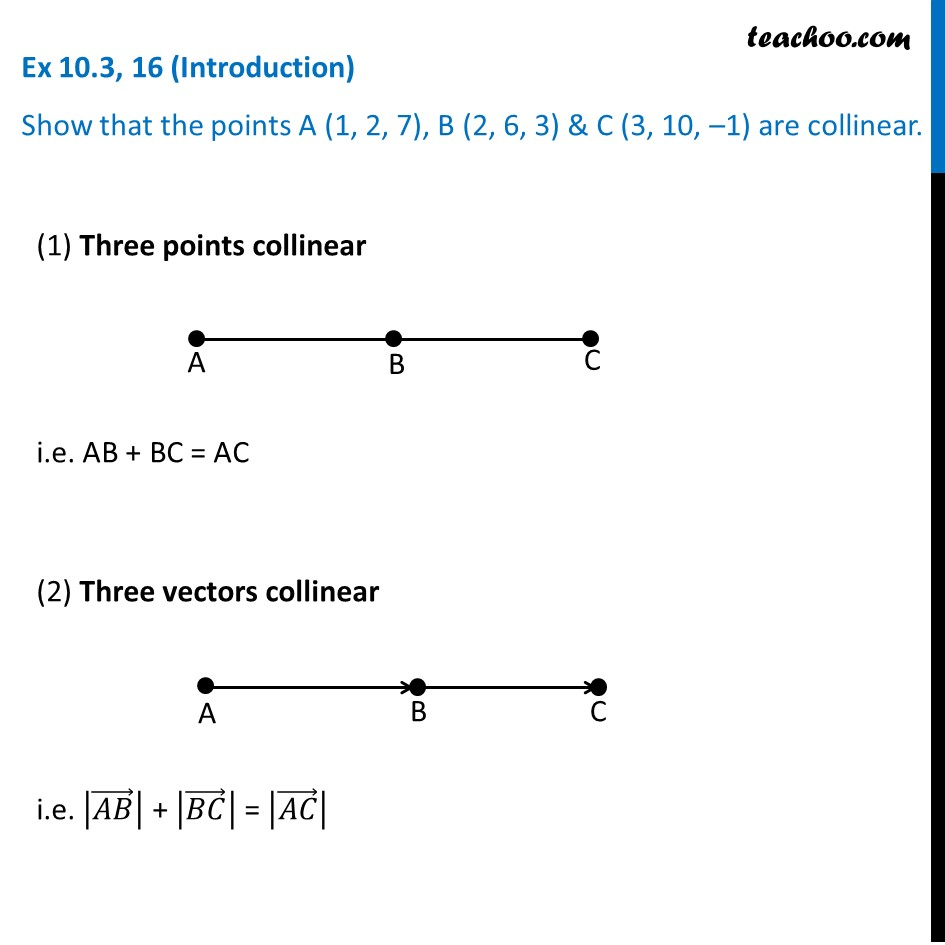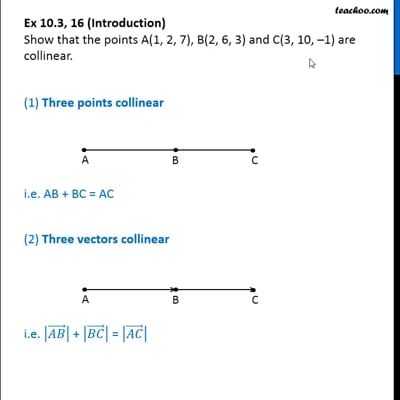This video is only available for Teachoo black users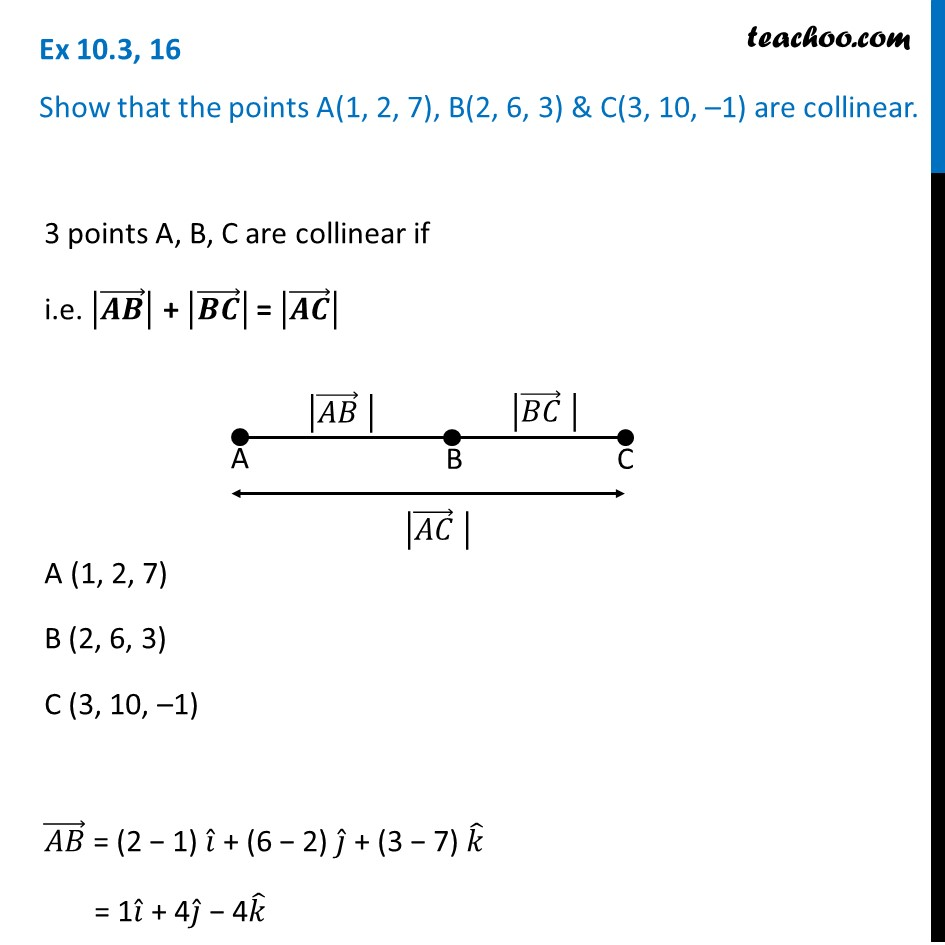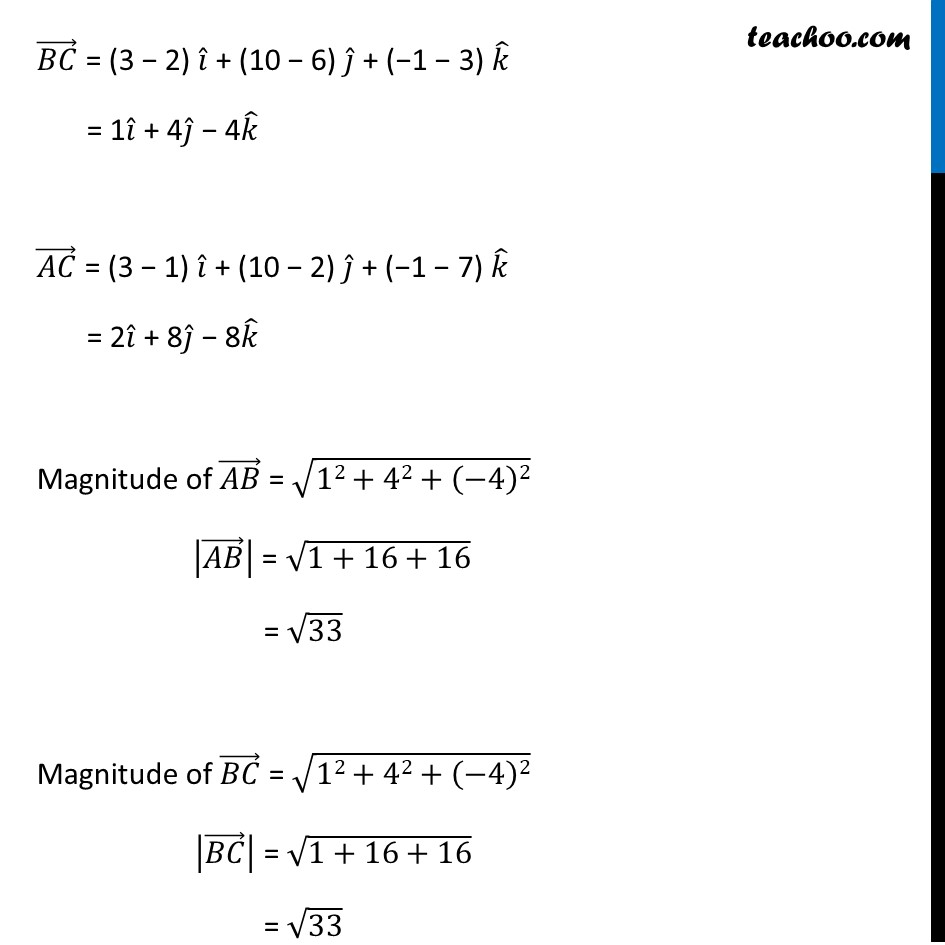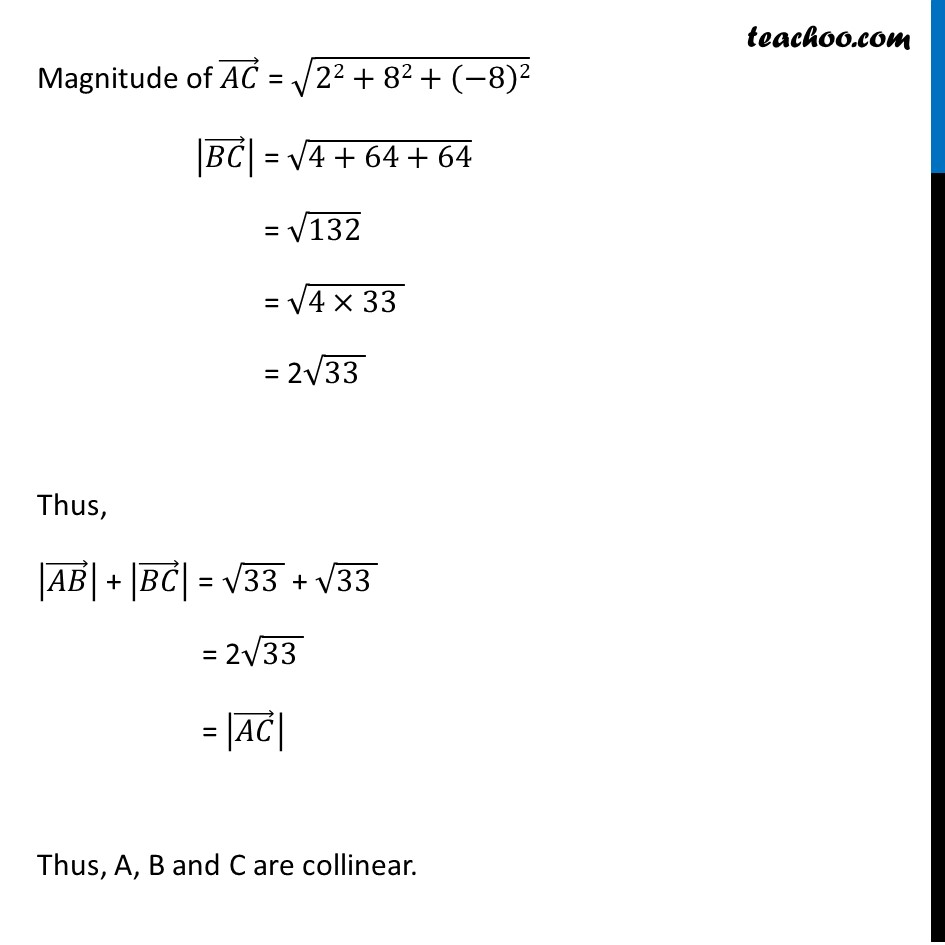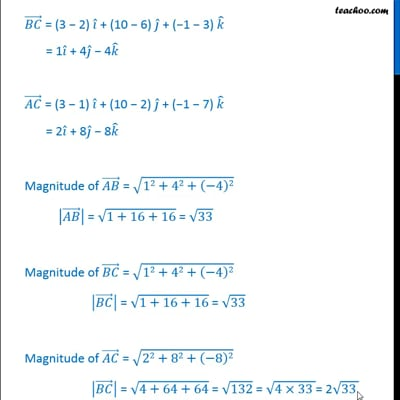This video is only available for Teachoo black users

Learn in your speed, with individual attention - Teachoo Maths 1-on-1 Class

### Transcript

Ex 10.3, 16 (Introduction) Show that the points A (1, 2, 7), B (2, 6, 3) & C (3, 10, –1) are collinear. (1) Three points collinear i.e. AB + BC = AC (2) Three vectors collinear i.e. |(𝐴𝐵) ⃗ | + |(𝐵𝐶) ⃗ | = |(𝐴𝐶) ⃗ | (𝐵𝐶) ⃗ = (3 − 2) 𝑖 ̂ + (10 − 6) 𝑗 ̂ + (−1 − 3) 𝑘 ̂ = 1𝑖 ̂ + 4𝑗 ̂ − 4𝑘 ̂ (𝐴𝐶) ⃗ = (3 − 1) 𝑖 ̂ + (10 − 2) 𝑗 ̂ + (−1 − 7) 𝑘 ̂ = 2𝑖 ̂ + 8𝑗 ̂ − 8𝑘 ̂ Magnitude of (𝐴𝐵) ⃗ = √(12+42+(−4)2) |(𝐴𝐵) ⃗ | = √(1+16+16) = √33 Magnitude of (𝐵𝐶) ⃗ = √(12+42+(−4)2) |(𝐵𝐶) ⃗ | = √(1+16+16) = √33 Magnitude of (𝐴𝐶) ⃗ = √(22+82+(−8)2) |(𝐵𝐶) ⃗ | = √(4+64+64) = √132 = √(4×33 ) = 2√(33 ) Thus, |(𝐴𝐵) ⃗ | + |(𝐵𝐶) ⃗ | = √(33 ) + √(33 ) = 2√(33 ) = |(𝐴𝐶) ⃗ | Thus, A, B and C are collinear.